Question

What will happen to the average speed of an object if the distance increases, but the time decreases?

1.thienan

Average speed will increase

Explanation:

First of all, average speed is given by;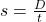s = average speed

D = Distance

t = time

From the above equation, we can see that average speed s, is directly proportional to distance D and inversely proportional to time t.

Case 1: Directly proportional means if variable A increases, variable B also increases and if variable B increases, variable A will also increase.

From the above equation, under the direct proportionality,

we have average speed and distance, this means as average speed increases, distance also increases.

The question tells us that distance increases which means average speed will also increase

Case 2: Indirectly proportional means if variable A increases, variable B will decrease and if variable B increases, variable A will decrease.

From the above equation, under indirect proportionality, we have average speed and time, this means if average speed inceases, time will decrease and vice versa.

The question tells us that time decreases, this means average speed will increase.

Conclusion: , we can see that the outcome of case 1 is in agreement with the outcome of case 2, in both cases, average speed increases.

For example: Let us assume that the initial distance was 10m and initial time was 5s, initial speed would be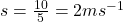Therefore, assuming distance is increased from 10m to 15m, and time decreases from 5s to 3s, the new speed will be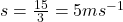We can see from the example that under the conditions of increased distance and decreased time, average speed increased from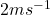to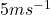Therefore, average speed will increase

2.